## Filters

Sort by :
Clear All
Q

Q.2 Draw a graph for the following

(ii)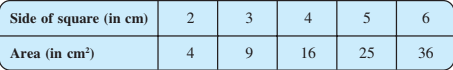Is it a linear  graph?

(ii) From above graph,we conclude that graph is not linear.

Q.2 Draw a graph for the following Is it a linear graph

(i)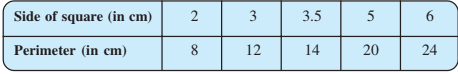(i) From the above graph ,we conclude that graph is linear.

Q.1 (c) Interest on deposits for a year .(iii) To get an interest of Rs 280 per year  how much money should be deposited ?

(c)  (iii) From the graph,to get an interest of Rs 280 per year  Rs 3500 money should be deposited.

Q.1 (c) Interest on deposits for a year(ii) Use the graph to find the interest on Rs 2500 for a year.

(c)  (ii) Using the graph, the interest on Rs 2500 for a year is Rs 200.

Q.1 (c) Interest on deposits for a year .(i) Does the graph pass through the origin?

(c)    Taking x axis as 1000 units = 1 box and y axis as 100 units = 1 box. (i)Yes, the graph pass through the origion.

Q.1 Draw the graphs for the following tables of values, with suitable scales on the axe.

Distance travelled by a car.

(b)(ii) What was the time when the car had covered a distance of 100 km since it’s star

(b)  (ii)From above graph we conclude that at 7:30 a.m.,  the car had covered a distance of 100 km since it’s start.

Q.1 Draw the graphs for the following tables of values, with suitable scales on the axes . Distance travelled by a car.

(b) (i)How much distance did the car cover during the period 7.30 a.m. to 8 a.m?(b)   (i)From the above graph,the distance the car cover during the period 7.30 a.m. to 8 a.m is

Q.1 Draw the graphs for the following tables of values, with suitable scales on the axes.
(a) Cost of apples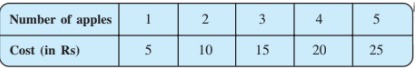(a) Cost of apples

Q. A bank gives 10% Simple Interest (S.I.) on deposits by senior citizens. Draw a graph to
illustrate the relation between the sum deposited and simple interest earned. Find from

Try a case of direct variation?

Taking a case of direct variation the graph is as shown below. Taking sum deposite as 100,200,300,500 ,1000.  and  10% Simple Interest (S.I.).

Q.1 In the above example, use the graph to find how much petrol can be purchased
for  Rs 800.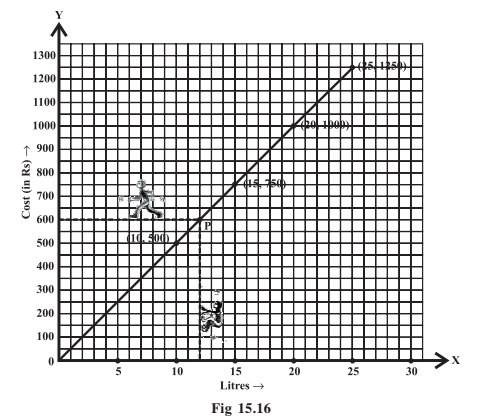From the graph given below we can say that for  Rs 800 we can buy 16 litres.

Q.4 State whether True or False. Correct
that are false.

(iii) The coordinates of the origin
are (0, 0).

(iii)True. The coordinates of the origin are (0, 0).

Q.4 State whether True or False. Correct that are false.

(ii) A point whose y coordinate is zero and x-coordinate is 5 will lie on
y-axis.

(ii) False.Point  does not lie on y-axis.A point whose y coordinate is zero and x-coordinate is 5 will not lie on y-axis.

Q.4 State whether True or False. Correct that are false.
(i) A point whose x coordinate is zero and y-coordinate is non-zero will
lie on the y-axis.

(i) True .A point whose x coordinate is zero and y-coordinate is non-zero will lie on the y-axis. =  here, y is variable.

Q.3 Write the coordinates of the vertices of each of these adjoining figures.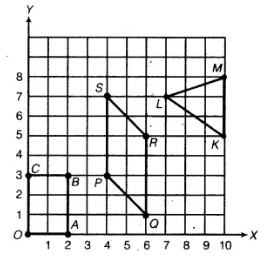The coordinates of vertices are: , ,, ,,, ,,

Q.2 Draw the line passing through (2, 3) and (3, 2). Find the coordinates of the points at
which this line meets the x-axis and y-axis.

As shown below is graph : The coordinates of the points at which this line meets the x-axis and y-axis are

Q.1 Plot the following points on a graph sheet. Verify if they lie on a line

(iii) K(2, 3), L(5, 3), M(5, 5), N(2, 5)

(iii) K(2, 3), L(5, 3), M(5, 5), N(2, 5) The graph is as shown :

Q.1 Plot the following points on a graph sheet. Verify if they lie on a line

(b) P(1, 1), Q(2, 2), R(3, 3), S(4, 4)

(b) P(1, 1), Q(2, 2), R(3, 3), S(4, 4) The graph is as shown :

Q.1 Plot the following points on a graph sheet. Verify if they lie on a line

(a) A(4, 0), B(4, 2), C(4, 6), D(4, 2.5)

(a) A(4, 0), B(4, 2), C(4, 6), D(4, 2.5)

(iv)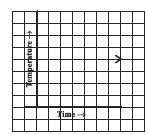(iv) Yes, it is possible i.e. same temperature at different time  is possible.

Q. 7Can there be a time-temperature graph as follows? Justify your answer.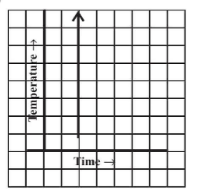(iii) No,this type of graph is not possible because different temperature at same time are not posiible.
Exams
Articles
Questions